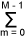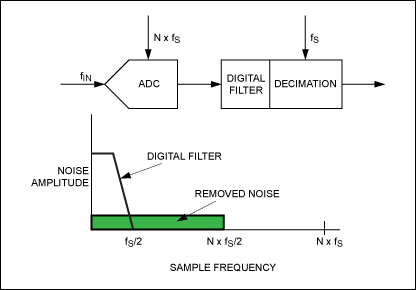# Understanding Noise, ENOB, and Effective Resolution in Analog-to-Digital Converters

### Abstract

Specifications such as noise, effective number of bits (ENOB), effective resolution, and noise-free resolution in large part define how accurate an ADC really is. Consequently, understanding the performance metrics related to noise is one of the most difficult aspects of transitioning from a SAR to a delta-sigma ADC. With the current demand for higher resolution, designers must develop a better understanding of ADC noise, ENOB, effective resolution, and signal-to-noise ratio (SNR). This application note helps that understanding.

A similar version of this article was published in Planet Analog on September 16, 2011.

One of the major trends for ADCs is the move toward higher resolution. The trend impacts a wide range of applications, including factory automation, temperature sensing, and data acquisition. The need for higher resolution is leading designers from traditional 12-bit successive approximation register (SAR) ADCs to delta-sigma ADCs with resolutions that reach 24 bits.

All ADCs have a certain amount of noise. That includes both input-referred noise, which is inherent to the ADC, and quantization noise, which is the noise generated while the ADC is converting. Specifications such as noise, effective number of bits (ENOB), effective resolution, and noise-free resolution in large part define how accurate an ADC really is. Consequently, understanding the performance metrics related to noise is one of the most difficult aspects of transitioning from a SAR to a delta-sigma ADC. With the current demand for higher resolution, designers must develop a better understanding of ADC noise, ENOB, effective resolution, and signal-to-noise ratio (SNR). This article helps that understanding.

### Higher Resolution and the Value of Delta-Sigma ADCs

In the past, a 12-bit SAR ADC was often good enough to measure a wide variety of signals and voltage inputs. If an application needed finer measurement, a gain stage or programmable gain amplifier (PGA) could be added in front of the ADC.

At 16 bits, a designer's choice is still primarily SAR ADCs, but also includes some delta-sigma ADCs. However, for designs that need more than 16 bits, delta-sigma ADCs are becoming much more prevalent. SAR ADCs are currently limited to 18 bits, while delta-sigma ADCs are expanding their presence at 18, 20, and 24 bits. There are other advantages to delta-sigma ADCs. Their price has dropped considerably in the last 10 years and they have become simpler to use and more widely understood.

### Effective Resolution

Effective resolution is defined in units of bits with the equation:

Effective resolution = log2 [full-scale input voltage range/ADC RMS noise]

Or perhaps more simply as:

Effective resolution = log2 [VIN/VRMS_NOISE]

Effective resolution should not be confused with ENOB, although they sound very similar. The most common methodology for measuring ENOB uses an FFT analysis of a sine-wave input to the ADC. The IEEE® standard 1057 defines ENOB as:

ENOB = log2 [full-scale input voltage range/(ADC RMS noise × √12)]

SINAD is defined as the signal-to-noise plus distortion ratio. SINAD and ENOB are used to measure the ADC's dynamic performance.

Therefore:

SINAD = [RMS input voltage/RMS noise voltage]

Where RMS noise = 1/M[EAVM(FM)^2].

Where EAVM = residual of XAVM, and XAVM(FM) is the averaged magnitude spectral component at a given discrete frequency after DFT.

Effective resolution and noise-free resolution measure the ADC's noise performance at essentially DC, where spectral distortion (THD, SFDR) is not factored.

Once the ADC's noise and input range are known, calculating effective resolution and noise-free resolution becomes simple.

The ADC's input voltage range is based on the reference voltage. If the ADC integrates a PGA, that must be factored into the voltage range as well. Some delta-sigma ADCs include PGAs to gain up small signals. The newest ADCs with PGAs often specify the noise as < 100nVRMS. While these noise figures look impressive compared to older ADCs, they are often based on a very small input range. This is because the small range will ultimately be amplified to fit a wider portion of the ADC's active range based on the reference voltage. So while the noise looks small for these ADCs with PGAs, the effective resolution and noise-free resolution may not be quite as good as ADCs without PGAs.

Consider a simple example. A 24-bit ADC with a PGA setting of 128 offers 70nVRMS noise with a reference voltage of 2.5V and an input range of ±VREF/PGA (±2.5V/128 = 39.1mV). The effective resolution is, therefore:

log2 [VIN/VRMS_NOISE] = log2 [39.1mV/70nV] = 19.1 bits

Using the same ADC with a PGA setting of 1, the noise rises to 1.53µVRMS. With an input range of 5V (±2.5V/1), the effective resolution becomes 21.6 bits.

The best practice is to check the ADC data sheet for the input range that you need.

### Noise-Free Resolution

Noise-free resolution uses the peak-to-peak voltage noise rather than the RMS noise. Noise-free resolution, also in bits, is defined by the equation:

Noise-free resolution = log2 [full-scale input voltage range/ADC peak-to-peak noise]

Noise-free resolution = log2 [VIN/VP-P_NOISE].

Noise-free resolution is also sometimes referred to as flicker-free resolution. Think of this in terms of a 5½- or 6½-digit multimeter in the lab. If the last digit on the display is stable and not flickering, the data output word is better than the noise level of the system.

Using a crest factor of 6.6 as an example, the peak-to-peak noise is 6.6x the RMS noise. As a result, the effective resolution is 2.7 bits higher than the noise-free resolution. Using the same noise and reference values above, the noise-free resolution is 18.9 bits.

### Noise-Free Counts

Noise-free counts is another metric that precision systems use to evaluate ADC performance. This is particularly true with an application like weigh scales, where 50,000 noise-free counts might be needed. This value can be calculated by converting the noise-free resolution into counts by a factor of 2N.

An example is a 10-bit ADC. Using the formula 210, an ideal 10-bit ADC has 1,024 noise-free counts. An ideal 12-bit ADC has 4,096 noise-free counts. Again, using the same noise-free resolution values above, that example would yield 218.9, or 489,178 noise-free counts.

One of the strengths of delta-sigma ADCs is their oversampling architecture. This means that the internal oscillator/clock is running at a much higher frequency than the output data rate, also referred to as the throughput rate. Some delta-sigma ADCs can vary the output data rate. This allows designers to optimize sampling for higher speeds with worse noise performance, or for lower speeds with more filtering, noise shaping (pushing the noise into the frequency band outside the area of measurement interest), and better noise performance. Many of the newest delta-sigma ADCs offer the effective resolution and noise-free resolution results in table form, making it easy to compare the trade-offs.

Table 1 shows an example ADC's data rate, noise, noise-free resolution (NFR), and effective resolution in both bipolar input modes and unipolar modes. The ADC is the MAX11200, a 24-bit device capable of measuring either bipolar (±VREF) or unipolar (0V to VREF) inputs. The MAX11200 operates from a single 2.7V to 3.6V supply, with the reference capable of being biased up to the supply. The bipolar values are based on the maximum input range of ±3.6V; the unipolar measurements are based on a 0V to 3.6V input range.

The MAX11200's internal oscillator can be programmed through the software for 2.4576MHz for 60Hz rejection at the lower data-rate settings, or for 2.048MHz for 50Hz rejection at lower data rates. At either data rate, the ADC noise is the same. Therefore, the resulting noise-free resolution and effective resolution values are consistent. An external oscillator can be applied for a 55Hz notch that gives good rejection at both 50Hz and 60Hz.

One key factor detailed in Table 1 is the bipolar effective resolution. This is limited to 24 bits maximum because the output data word is 24 bits long. At the three slowest data-rate settings, the ADC's noise level is low enough that the effective resolution is better than 24 bits if the ADC were to output more than 24 bits of data on the serial interface.

Effective resolution is always 2.7 bits better than the noise-free resolution, unless you are limited by the data output word.

 Data Rate (sps) ADC Noise (µVRMS) Bipolar Noise-Free Resolution (Bits) Bipolar Effective Resolution (Bits) Unipolar Noise-Free Resolution (Bits) Unipolar Effective Resolution (Bits) * ** 1 0.83 0.21 22.3 24.0 21.3 24.0 2.5 2.08 0.27 22.0 24.0 21.0 23.7 5 4.17 0.39 21.4 24.0 20.4 23.1 10 8.33 0.57 20.9 23.6 19.9 22.6 15 12.5 0.74 20.5 23.2 19.5 22.2 30 25 1.03 20.0 22.7 19.0 21.7 60 50 1.45 19.5 22.2 18.5 21.2 120 100 2.21 19.0 21.7 18.0 20.7 *Internal oscillator is 2.4576MHz for 60Hz rejection. **Internal oscillator is 2.048MHz for 50Hz rejection.

### Noise Shaping and Filtering for Lower Noise and Better Resolution

Besides oversampling, noise shaping allows delta-sigma ADCs to achieve the low noise and high precision shown in Table 1. This is illustrated in Figures 1 through 3. Figure 1 shows a standard ADC's quantization noise. Figure 2 details an ADC that includes oversampling, a digital filter, and decimation. The large majority of ADC cores that use oversampling are delta sigma. Oversampling by a factor of N spreads the noise over a wider frequency band, while the digital (sinc) filter removes a good portion of the noise.Figure 3 details a delta-sigma modulator with the same blocks as Figure 2, plus noise-shaping. By pushing the noise disproportionately to higher frequencies, the noise in the frequency band of interest becomes ultra low. Techniques like this allow delta-sigma ADC manufacturers to achieve < 1µVRMS noise figures.### Conclusion

With their oversampling capability and inherently low noise, delta-sigma ADCs are an excellent design choice for systems that require higher resolution. As designers must resolve even smaller signals, a firm understanding of ADC noise, effective resolution, ENOB, and noise-free resolution becomes integral to choosing the right ADC solution.